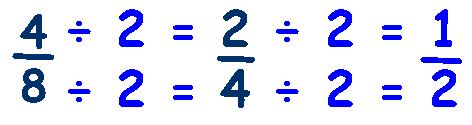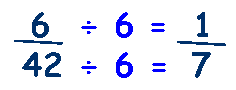# Simplifying Fractions

We know that fractions can look different but have the same value (Equivalent Fractions) so 25/50 is the same as 1/2. Which one is easier to work with?

It is much easier to understand half of something than try to work out
what twenty five fiftieths are.

We need to be able to modify a fraction so that it is easier to work with - to make it simpler, which is why we learn to Simplify Fractions.

## Remember that with a fraction, whatever we do to the top (numerator), we must do to the bottom (denominator).

To simplify a fraction, we try dividing the top and bottom until we cant go any further.

Try to divide by '2' (if both numbers are even then it will divide by 2),
if not try 3 and then 5, then 7 etc.

Look at this fraction, 2/4, both top and bottom will divide by 2,Try this fraction, 4/8,we can divide by 2, and then by 2 again.

Of course you may have noticed that we could have divided the numerator and denominator by 4 and achieved the same result in just one step!

Lets try another example, say 6/42, divide both by 2,Can we simplify further? Yes, divide both by 3,Of course you may have noticed that 6 and 42 are in the 6 times table, so we could have divided by 6 and achieved the same result in just one step!

_____________________________________________

An alternative method involves using our knowledge of factors to find the Highest Common Factor that is shared between the numerator and denominator and then dividing both numerator and denominator by that number.

Looking at the example above - numerator is 6 and denominator is 42

Factors of 6 are: 1, 2, 3, and 6

Factors of 42 are: 1, 2, 3, 6, 7, 14, 21 and 42

The Highest Common Factor is 6, so divide both by 6,Practice simplifying fractions with these worksheets

or these from Dr. Mikes Maths Games

Simplifying Fractions Quiz